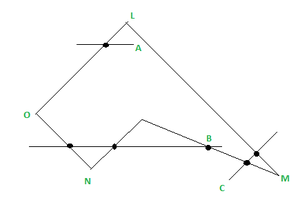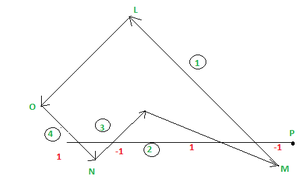# Even Odd method & Winding number Method-Inside & Outside Test of a Polygon

• Difficulty Level : Easy
• Last Updated : 06 Jul, 2021

Introduction :
A polygon may be represented as a number of line segments connected, end to from a closed figure.
Polygons can be represented in two ways –
(i) outline from using move commands and
(ii) as a solid objects by setting the pixels high inside the polygon including pixels on the boundary.

To determine a point lies inside a polygon or not, in computer graphics, we have two methods :
(a) Even-Odd method (odd-parity rule)
(b) Winding number Method-Inside

Even-Odd method :
Constructing a line segment between the point (P) to be examined and a known point outside the polygon is the one way to determine a point lies inside a polygon or not. The number of times the line segment intersects the polygon boundary is then counted. The point (P) is an internal point if the number of polygon edges intersected by this line is odd; otherwise, the point is an external point.

In the figure, the line segment from ‘A’ crosses single edge & hence point A is inside the polygon. The point B is also inside the polygon as the line segment from B crosses three (odd) edges. But point C is outside the polygon it  as the line segment from C crosses two (even) edges.Polygon

But this even-odd test fails when the intersection point is a vertex.
To handle this case, we have to make few modifications.
We must look at the other end points of the two segments of a polygon which meet at this vertex. If these points lies on the same side of the constructed line A’P’, then the intersection point counts as an even number of intersection. But if they lie on the opposite side of constructed line AP, then the intersection points counts as a single intersection.Polygon

As we can see the line segment A’P’ intersects at M which is a vertex and L & Z are the other end points of the two segments meeting at M. L & Z lie on same side of the line segment A’P’, so the count is taken as even.

Winding Number Method :
There is another alternative method for defining a polygons’ interior point is called the winding number method. Conceptually, one can stretch a piece of elastic between the point (P) to be checked and the point on the polygon boundary.
Treat that, elastic is tied to point (P) to be checked firmly and the other end of the elastic is sliding along the boundary of the polygon until it has made one complete circuit. Then we check that how many times the elastic has been wound around the point of intersection. If it is wound at-least once, point is inside. If there is no net winding then point is outside.

In this method, instead of just counting the intersections, we give each boundary line crossed a direction number, and we sum these directions numbers. The direction number indicates the direction of the polygon edge was drawn relative to the line segment we constructed for the test.

Example : To test a point (xi, yi), let us consider a horizontal line segment y = yi which runs from outside the polygon to (xi, yi). We find all the sides which crossed this line segment.
Now there are 2 ways for side to cross, the side could be drawn starting below end, cross it and end above the line. In this case we can give direction numbers – 1, to the side or the edge could start above the line & finish below it in this case, given a direction 1. The sum of the direction numbers for the sides that cross the constructed horizontal line segment yield the “Winding Number” for the point.

If the winding number is non-zero , the point is interior to polygon, else, exterior to polygon.Polygon

In the above figure, the line segment crosses 4 edges having different direction numbers : 1, -1, 1& -1 respectively, then :
Winding Number = 1 + (-1) + 1 + (-1) = 0
So the point P is outside the Polygon. The edge has direction Number -1 because it starts below the line segment & finishes above. Similarly, edge has direction Number +1 because it starts from above the line segment & finishes below the line segment (See the directions in the figure).

My Personal Notes arrow_drop_up
Recommended Articles
Page :